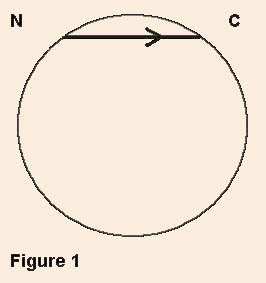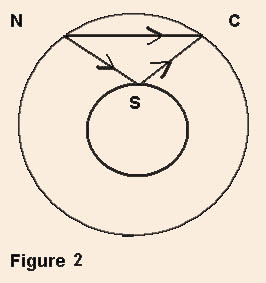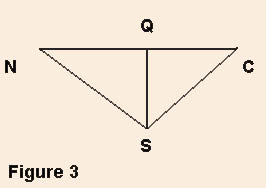Keyah Math Study 10, Level 3
Mathematical Conte
nt : Basic algebra (distance, rate and time); geometry; Snell’s law

# Exploring the Interior of the Earth: ExercisesIn the following exercises, refer to Figure 1 (left), and round all answers to 1 decimal place.

 Information: You found the radius of the Earth to be 6,490 kilometers using the methods of Eratosthenes. Actually, the earth is not quite spherical, it bulges some at the equator, but the average radius of the Earth is 6,371 km, so that's what we'll use for this exploration.

An earthquake occurs at the indicated location labeled N on Figure 1. A seismograph located at a station labeled C which is 80 kilometers from N records the first seismic wave 12.3 seconds after the earthquake occurs.

 Question 1: Using the known travel time and distance, compute the velocity of the seismic wave.

ASSUME: Since this is the first wave that reaches station C, we assume that this wave is the one that traveled in a straight line (shortest distance) from N to C. We assume that the distance, 80 km, from N to C along the surface of the Earth is approximately equal to the straight line distance from N to C.

A second wave arrives at station C 18.8 seconds after the earthquake occurs.

 Question 2: Find the distance this second wave traveled. (Use the information about the second wave and the velocity you have computed.)

 Question 3: Can you guess what path that this second wave traveled?If you guessed that the wave must have bounced off some barrier inside the Earth, then you are correct! See Figure 2.

Next we will find the depth of this barrier. See Figure 3.Information: Laws of physics tell us: the seismic wave bounces off the barrier at the point labeled S at the same angle that it hits the barrier. Also, from geometry, we know these facts: the sides NS and SC have the same length; the line from S to Q divides the triangle NCS into two right triangles NQS and CQS that are the same size.
 Question 4: Compute the depth SQ of the barrier. (Use the information together with your previous answers to compute SQ, the depth of the barrier).

ASSUME: Here again, we assume that the straight line from N to C is the same as the very slightly curved line from N to C, so the length of QS is the same as the very slightly longer distance from S to the surface of the Earth.)

 Question 5: Compute the radius of the interior barrier.

ASSUME: The Earth and this newly discovered interior "barrier" are both sphericalThis is one way to confirm that the Earth is layered, i.e., there's some inner core that's thick enough to reflect seismic waves!

This material is based upon work supported by the National Science Foundation under Grant GEO-0355224. Any opinions, findings, and conclusions or recommendations expressed in this material are those of the authors and do not necessarily reflect the views of the National Science Foundation.